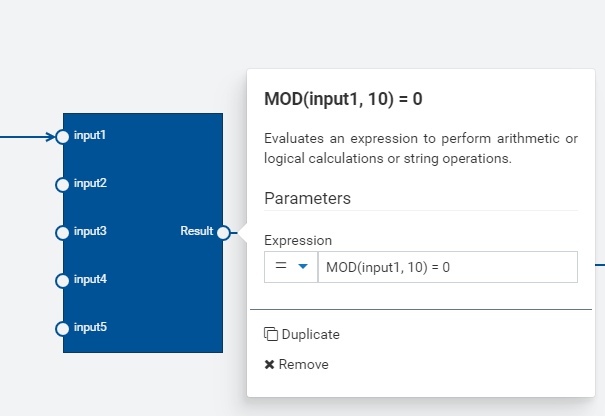# Expression block in the analytic builder model?"I’m trying to create an expression block in Analytics Builder that checks whether a given input value is divisible by 10 or not. I’ve tried using the ‘%’ operator, but it’s causing a runtime error. Can you suggest an alternative expression or function that I can use to perform this check? Thanks

MOD(input1, 10) = 0
input1 / 10 = floor(input1 / 10)
IF(input1 % 10 = 0, true, false)
(input1 / 10).fractionalPart() == 0
input1 - (input1 / 10).round() * 10 == 0

Have two expression blocks, one that divides the input by 10, the next then uses input1.fractionalPart() to pass the value after the decimal point. Then test it for 0. If it is 0 then the original value was divisible by 10.

SOLVED with :
(input1 / 10).fractionalPart() = 0

This topic was automatically closed 180 days after the last reply. New replies are no longer allowed.